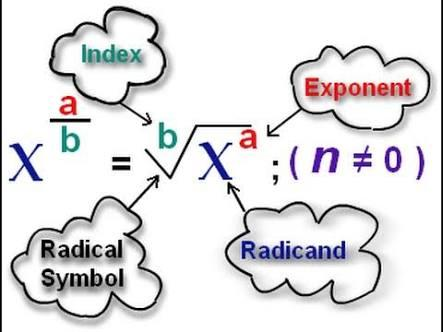10 Questions | Total Attempts: 188SettingsIn as much as you know a bit about the topic, you'll be completely fine. If you don't, then you'll learn more.

• 1.
What's the most crucial symbol used under this topic?
• A.

Division

• B.

Square roots

• C.

Square of a number

• D.

Cube of a numbee

• 2.
What represents square root?
• A.

• B.

π

• C.

°

• D.

|

• 3.
What is a rational exponent?
• A.

A number

• B.

A number greater than 10

• C.

A whole number

• D.

A fraction

• 4.
What's the relationship between rational exponents and radicals?
• A.

Rational exponents and radicals are progressive equations used in resolving bigger problems

• B.

They're in the same form

• C.

Rational exponents and radicals are different ways of expressing the same thing

• D.

They are separate entities that cannot work together

• 5.
Numerators in fractional exponents start from where?
• A.

0

• B.

1

• C.

4 above only

• D.

1.5

• 6.
Rewriting a radical entails one of the following
• A.

Root is multiplied by 2

• B.

Root is squared

• C.

Radicand becomes numerator while root becomes denominator

• D.

Radicand is multiplied by the root

• 7.
Where, in the following do you use fractional exponents?
• A.

None

• B.

Calculus

• C.

Factorization

• D.

Trigonometry

• 8.
• A.

They limit calculations a lot

• B.

They provide different ways to solve certain/specific problems

• C.

They don't make use of numerators or denominators

• D.

They leave remainders

• 9.
Which number correctly represents cube root?
• A.

3

• B.

2

• C.

4

• D.

2.8 (approximately 3)

• 10.
• A.

÷

• B.

|

• C.

°

• D.

Related TopicsBack to top